Previous: 3.3.3 Electronic Stopping Process Up: 3.3.3 Electronic Stopping Process Next: 3.3.3.2 Effective Charge of

### 3.3.3.1 Energy Loss of a Point Charge in a Free Electron Gas

A solution of this problem was already given by Lindhard . The energy loss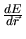of the projectile is determined by the force resulting from the electric field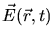which is generated in the plasma by the penetrating charged particle with a charge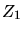moving with the velocity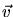. For his solution Lindhard applied the linear response theory.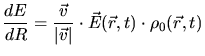(3.100)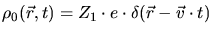(3.101)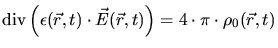(3.102)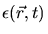is the linear dielectric response function of the medium on the charge.

This set of differential equations can be solved by Fourier transformation  (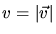).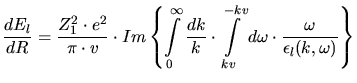(3.103)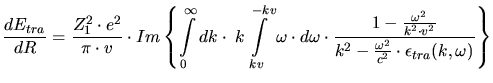(3.104)

The contributions to the energy loss by the transversal electric field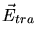and the longitudinal electric field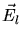are treated separately, which requires the definition of a transversal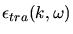(Fourier transform of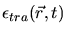) and a longitudinal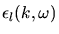(Fourier transform of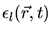) dielectric response function.andare defined in Fourier space via the scalar potentialand vector potential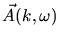, describing the electromagnetic field as shown in (3.105) and (3.106), and the Maxwell equations.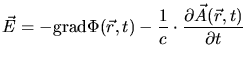(3.105)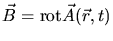(3.106)is defined by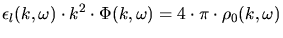(3.107)

whileis defined by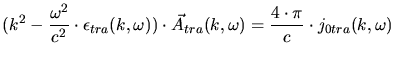(3.108)

The transversal field and the transversal current density are characterized by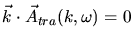(3.109)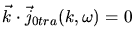(3.110)

The total energy loss is a sum of the longitudinal and the transversal contribution, respectively. The transversal contribution is negligible since the particle velocity is non-relativistic . This requirement holds for typical ion implantation conditions.

Lindhard derived an expression for the longitudinal dielectric response functionby applying a self-consistent treatment of the electron gas. He calculates the charge density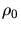and the current densityinduced in the plasma by the forces resulting from the scalar potential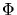and the vector potential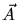. These induced sources produce an electro-magnetic field which acts on the electron gas. Therefore the electro-magnetic field must be self-consistent with the induced sources. A quantum-mechanical calculation of this problem results in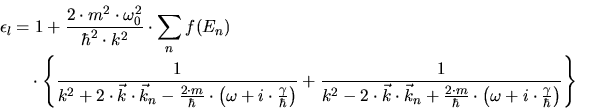(3.111)

with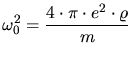(3.112)

being the classical resonance frequency of the gas.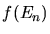is the electron density distribution function,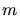is the electron mass,is the energy,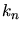the wave vector of the electron in the nth state and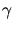is a small damping factor. By applying this dielectric response function to (3.103) Lindhard derived the energy loss in an electron gas described by the Fermi distribution in the ground state with a maximum energy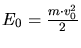.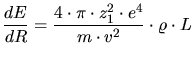(3.113)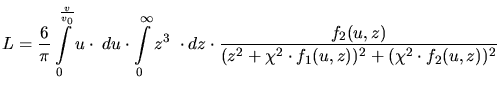(3.114)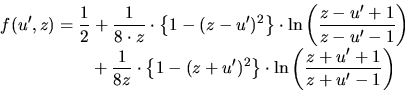(3.115)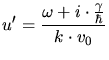(3.116)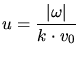(3.117)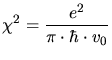(3.118)

Assuming a small damping factorand a small particle velocity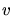, (3.113) can be simplified to an electronic stopping equation which is proportional to velocity.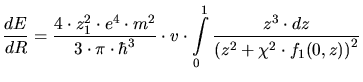(3.119)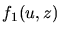is the real part of (3.115), while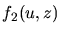is the imaginary part.

A. Hoessiger: Simulation of Ion Implantation for ULSI Technology